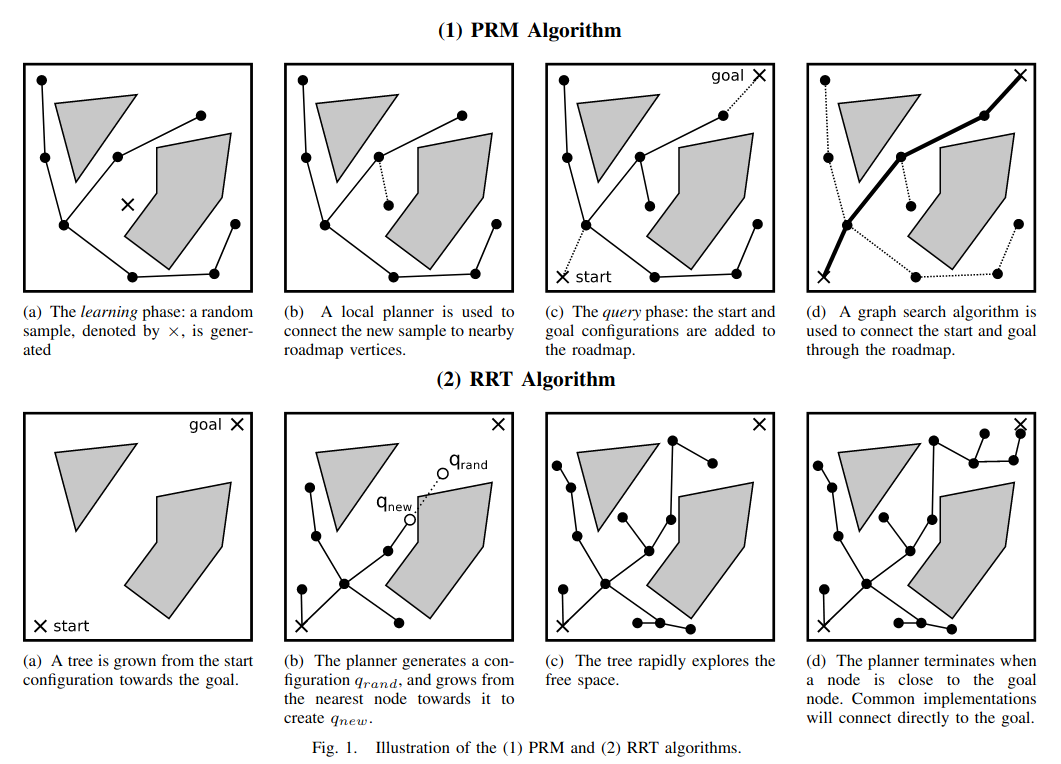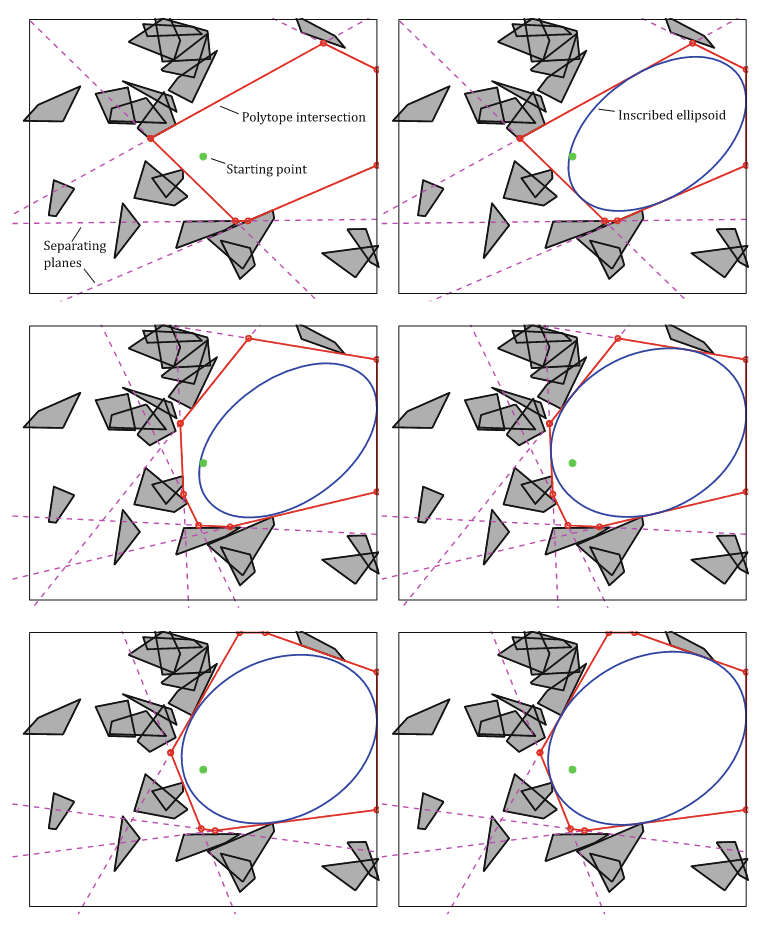### Reimplementing IRIS (Computing Large Convex Regions of Obstacle-Free Space Through Semidefinite Programming)

I've just begun my studying towards my PhD in computer science at MIT, and am working in the Robot Locomotion Group. This group has developed a series of projects that use formal mathematical optimization for tasks in motion planning. Because I'm interested in this area of research, I've been reading several of their papers; to better understand these papers, I've endeavored to reimplement some of their work.

The two specific algorithms I'm interested in are close companions: Iterative Regional Inflation by Semidefinite programming (IRIS) can be used to find large convex regions of collision free space, and shortest paths in Graphs of Convex Sets (GCS) can be used for motion planning when the configuration space is described as the union of convex sets. I've attempted to reimplement these algorithms for simple, toy problems.

Disclaimer: the intent is not to build a codebase for future use, since the Drake toolbox has mature implementations of both IRIS and GCS.

In this blog post, I'll give a brief overview of the IRIS paper, and then discuss my experiences reimplementing it. In a following blog post (which is yet to be written), I'll do the same for GCS. I have also made my code available on github, with links included at the end of this post.

## An Overview of IRIS

A major problem in motion planning is describing the set of collision-free points in some configuration space. (For brevity, this set is referred to as "C-Free".) For example, sampling-based planners such as RRT and PRM find a number of individual, collision-free configurations, and then connect proximal ones to build a graph structure.(Figure taken from Recent progress on sampling based dynamic motion planning algorithms, Short et. al.)

However, these algorithms do present drawbacks. Although the individual points are certified to be collision-free, doing so for all points along an edge is challenging. Another problem is that the number of sample points required to cover a configuration space (with a fixed density) grows exponentially with the dimension of that space.

The IRIS algorithm presents an alternative method for describing C-Free. IRIS constructs large convex collision-free subsets of configuration space. The convexity of the set leads to a valuable property: for any two points in a convex set, the entirety of the line segment connecting them is also contained that set. Thus, it's tractable to certify an entire trajectory as collision free, as opposed to just individual points along the trajectory.

IRIS constructs subset of C-Free by picking an initial "seed" point, and then attempting to find the largest collision-free convex polytope around that seed point. Because finding the volume of a convex polytope is #P-Hard (when represented as a halfspace intersection), the volume of the largest inscribed ellipsoid is used as a proxy. Thus, IRIS is formualted as maximizing the volume of an ellipse, contained within a halfspace intersection, such that those halfspaces separate the ellipse from all obstacles. This optimization problem is not convex, but it is biconvex in the decision variables defining the halfspaces and the ellipse; in practice, it can be efficiently solved by alternatingly fixing one set of variables and optimizing over the other set. In effect, IRIS switches between pushing the halfspace boundaries away from the ellipse (the "SeparatingHyperplanes" step), and growing the ellipse within the halfspace boundaries (the "InscribedEllipsoid" step).Figure 3 from Computing Large Convex Regions of Obstacle-Free Space Through Semidefinite Programming (Deits and Tedrake)

## Implementing IRIS

I implemented IRIS in Python, and applied it to a simple problem in 2D space. To generate a random world, I scatter a number of points randomly, and then compute an alpha shape. This generates random obstacles, and implicitly decomposes them into a set of simplices. Once the world is generated, I open a matplotlib visualization interface, and prompt the user to select seed points for the IRIS algorithm. Once a seed point has been selected, I visualize each half step of the IRIS algorithm separately.

Although the polytopic regions are represented by their halfspaces, it's easier to visualize them in terms of their vertices. I use the scipy HalfspaceIntersection class (an interface for the qhull library) to compute the vertices of intersection. These vertices may not be ordered, which is necessary for rendering a polygon. But the convexity of the region makes sorting the vertices straightforward: one simply needs to sort them based on their bearing relative to their centroid.

Of the two alternating steps taken by IRIS, the InscribedEllipsoid step is the simpler of the two. The optimization problem is clearly formulated in equation 10 of the IRIS paper, and only a few lines of code are required to define and solve the optimization problem. (In all instances, I use CVXPY for optimization.)

The other step taken by IRIS, SeparatingHyperplanes, primarily relies on several subroutines. This process requires every obstacle be separated from the ellipsoid, but handling nearby obstacles first is much more efficient -- a hyperplane that separates the ellipsoid from a nearby obstacle can also separate it from more distance obstacles. The "ClosestObstacle" routine simply entails computing the distance from the ellipse to each obstacle vertex, and selecting the closest one. Then, the "ClosestPointOnObstacle" routine computes the actual point on the obstacle which is closest to the ellipsoid by solving a quadratic program. Finally, the "TangentPlane" routine computes the separating hyperplane, which is a simple, closed-form computation.

Here is a video of my implementation:

## Conclusion

I've published my code for IRIS online in this git repository, so you can download it and try it out for yourself. I'm planning to write a blost post on my experience reimplementing GCS soon, but for now, you can find the code for that in this git repository.